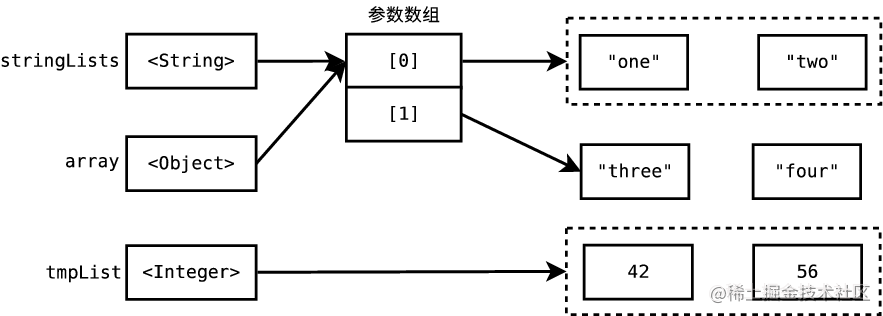# 引入泛型之前

``````List doubleList = new LinkedList();
Double d = (Double) doubleList.iterator().next(); //类型强制转换

doubleList中存储一个Double类型的值, 但是List并不能阻止我们往里面再添加一个String类型

# 引入泛型之后

``````List<Double> doubleList = new LinkedList<Double>();
Double d = doubleList.iterator().next();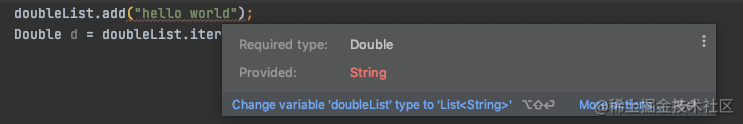# 深入探索泛型类

## 泛型的概念

`类型参数`:是一对尖括号之间以逗号分隔的类型参数名列表。

## 泛型类型遵循语法

``````class identifier<formalTypeParameterList>{

}

interface identifier<formalTypeParameterList>{

}

interface Map<K,V> {//多个用逗号分隔
}

## 类型参数命名原则

Java 编程约定要求类型参数名称为单个大写字母，例如 E 表示元素，K 表示键，V 表示值，T 表示类型。避免使用像A,B,C这样没有意义的名称。

List < E > 表示一个元素列表，但是 List < B > 的意思是什么呢？

## JAVA支持的实际类型的参数有哪些

• 类型参数: 类型参数 传递给 类型参数
``````class Container<E> {
Set<E> elements; //E传给E
}

• 具体类:传递具体的类

• 参数化类:传递具体的参数化类

• 数组类型: 传递数组

• 通配符: 使用问号(?)传递

# 声明和使用泛型

## 定义泛型的例子

``````class Container<E>  //也可以使用实际类型的参数
{
private E[] elements;
private int index;
Container(int size)
{
elements = (E[]) new Object[size];
//本例中我们传入的是String,将Object[]转化为String[]返回
index = 0;
}
{
elements[index++] = element;
}
E get(int index)
{
return elements[index];
}
int size()
{
return index;
}
}
public class GenDemo
{

public static void main(String[] args)
{
Container<String> con = new Container<String>(5);//使用String传给E,指定E为String类型的
for (int i = 0; i < con.size(); i++)
System.out.println(con.get(i));
}
}

## 指定类型参数的泛型

Container < E > 中的 E 为无界类型参数,通俗的讲就是什么类型都可以,可以将任何实际的类型参数传递给 E . 例如，可以指定 Container < Student > 、 Container < Employee > 或 Container < Person >

## 通过指定上限来限制传入的类

``````class Employees<E extends Employee>

## 指定多个类型限制

``````abstract class Employee
{
private BigDecimal hourlySalary;
private String name;
Employee(String name, BigDecimal hourlySalary)
{
this.name = name;
this.hourlySalary = hourlySalary;
}
public BigDecimal getHourlySalary()
{
return hourlySalary;
}
public String getName()
{
return name;
}
public String toString()
{
return name + ": " + hourlySalary.toString();
}
}
class Accountant extends Employee implements Comparable<Accountant>
/*
Comparable < Accountant > 表明Accountant可以按照自然顺序进行比较
Comparable 接口声明为泛型类型，只有一个名为 t 的类型参数。

*/

{
Accountant(String name, BigDecimal hourlySalary)
{
super(name, hourlySalary);
}
public int compareTo(Accountant acct)
{
return getHourlySalary().compareTo(acct.getHourlySalary());
}
}

class SortedEmployees<E extends Employee & Comparable<E>>

//第一个必须为class 之后的必须为interface
{
private E[] employees;
private int index;
@SuppressWarnings("unchecked")
SortedEmployees(int size)
{
employees = (E[]) new Employee[size];
int index = 0;
}
{
employees[index++] = emp;
Arrays.sort(employees, 0, index);
}
E get(int index)
{
return employees[index];
}
int size()
{
return index;
}
}
public class GenDemo
{
public static void main(String[] args)
{
SortedEmployees<Accountant> se = new SortedEmployees<Accountant>(10);
for (int i = 0; i < se.size(); i++)
System.out.println(se.get(i));
}
}

# 下界和泛型参数

``````class Scratch_12{
public static void main(String[] args) {
{
List<String> directions = new ArrayList();
printList(directions);
}

}
static void printList(List<Object> list)
{
Iterator<Object> iter = list.iterator();
while (iter.hasNext())
System.out.println(iter.next());
}
}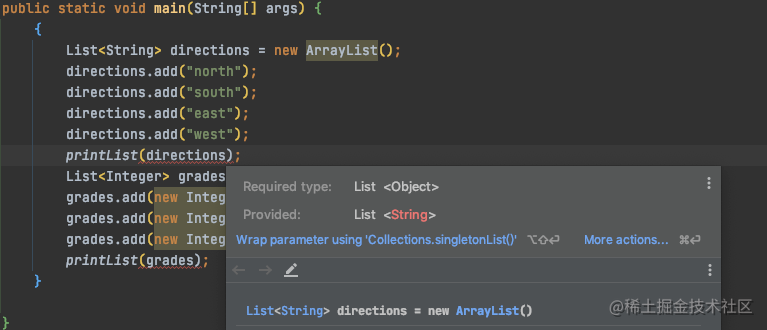For a given subtype x of type y, and given G as a raw type declaration, G< x > is not a subtype of G < y >.

``````List<String> directions = new ArrayList<String>();
List<Object> objects = directions;
String s = objects.get(0);

## 使用通配符来解决问题

``````class Scratch_13{
public static void main(String[] args) {
List<String> directions = new ArrayList<String>();
printList(directions);
}
static void printList (List < ? > list)
{
Iterator<?> iter = list.iterator();
while (iter.hasNext())
System.out.println(iter.next());
}

}

# 深入探索泛型方法

``````void copy(List<?> src, List<?> dest, Filter filter)
{
for (int i = 0; i < src.size(); i++)
if (filter.accept(src.get(i)))
}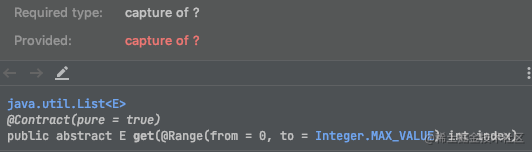< ？ >意味着任何类型的对象都可以是列表的元素类型，并且源元素和目标元素类型可能是不兼容的

## 指定类型上下界

``````void copy(List<? extends String> src, List<? super String> dest, Filter filter)
{
for (int i = 0; i < src.size(); i++)
if (filter.accept(src.get(i)))
}

## 使用泛型方法完全解决这个问题

``````<formalTypeParameterList> returnType method(param)

``````public class Demo
{
public static void main(String[] args)
{
{
Integer.valueOf(96),
Integer.valueOf(95),
Integer.valueOf(27),
Integer.valueOf(100),
Integer.valueOf(43),
Integer.valueOf(68)
};

for (int i = 0; i < gradeValues.length; i++){
}

for (int i = 0; i < failedGrades.size(); i++){
}

}

static <T> void copy(List<T> src, List<T> dest, Filter<T> filter)
{
for (int i = 0; i < src.size(); i++)
if (filter.accept(src.get(i)))
}
}
interface Filter<T>
{
boolean accept(T o);
}

# 泛型和类型推断

Java 编译器包含类型推断算法，用于在实例化泛型类、调用类的泛型构造函数或调用泛型方法时识别实际的类型参数。

## 泛型类实例化

``````Map<String, Set<String>> marbles = new HashMap<String, Set<String>>();

``````Map<String, Set<String>> marbles = new HashMap<>();//使用<>替换<String, Set<String>>

``````Map<String, Set<String>> marbles = new HashMap();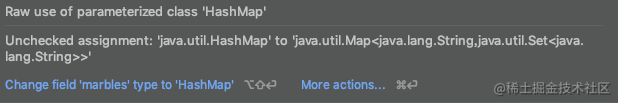# 泛型构造函数

``````public class Box<E>
{
public <T> Box(T t)
{
// ...
}
}

``````new Box<Marble>("Aggies");

``````new Box<>("Aggies");

# 泛型方法调用

``````//copy是静态方法 我们可以使用class.methodName的方式调用它

``````new Demo().<Integer>copy(grades, failedGrades, grade -> grade <= 50);

# 类型擦除

## 举例说明

ArrayList< String > () 和 ArrayList< Integer > ()

``````public class ErasedType {

public static void main(String[] args) {

Class c1 = new ArrayList<String>().getClass();
Class c2 = new ArrayList<Integer>().getClass();

// output：true
System.out.println(c1 == c2);
}
}

System.out.println(Arrays.toString(c1.getTypeParameters()));
// output：[E]
System.out.println(Arrays.toString(c2.getTypeParameters()));
// output：[E]

# 堆污染( heap pollution)

## 堆污染示例

``````  import java.util.Iterator;
import java.util.Set;
import java.util.TreeSet;
public class Scratch_15
{
public static void main(String[] args)
{
Set s = new TreeSet<Integer>();
Set<String> ss = s;            // unchecked warning Unchecked assignment: 'java.util.Set' to 'java.util.Set<java.lang.String>'
s.add(42);    // unchecked warning Unchecked call to 'add(E)' as a member of raw type 'java.util.Set'
Iterator<String> iter = ss.iterator();
while (iter.hasNext())
{
String str = iter.next();   //throw ClassCastException
System.out.println(str);
}
}
}
/*

Exception in thread "main" java.lang.ClassCastException: java.lang.Integer cannot be cast to java.lang.String
at Scratch_15.main(scratch_15.java:17)
*/

• 第一个未检查的警告:变量 ss 具有参数化类型 Set < string > 。当 s 引用的 Set 被分配给 ss 时，编译器会生成一个未检查的警告。它因为编译器不能确定 s 引用 Set < string > 类型。结果就是堆污染
• 第二个未检查的警告:由于泛型擦除,编译器也不能确定变量 s 是指 Set < string > 还是 Set < integer > 类型,这时就会产生 unchecked warning ，自然就会发生 堆污染了

# @SafeVarargs的用法

@SafeVarargs在JDK 7中引入，主要目的是处理可变长参数中的泛型，此注解告诉编译器：在可变长参数中的泛型是类型安全的。可变长参数是使用数组存储的，而数组和泛型不能很好的混合使用 简单的说，数组元素的数据类型在编译和运行时都是确定的，而泛型的数据类型只有在运行时才能确定下来，因此当把一个泛型存储到数组中时，编译器在编译阶段无法检查数据类型是否匹配，因此会给出警告信息：存在可能的“堆污染”(heap pollution)，即如果泛型的真实数据类型无法和参数数组的类型匹配，会导致ClassCastException异常。

``````import java.util.ArrayList;

public class SafeVarargsTest {
public static void main(String[] args) {
ArrayList<Integer> a1 = new ArrayList<>();
showArgs(a1, 12);
}

//@SafeVarargs
public static <T> void showArgs(T... array) {
for (T arg : array) {
System.out.println(arg.getClass().getName() + ":" + arg);
}
}

}

``````\$ javac -Xlint:unchecked SafeVarargsTest.java
SafeVarargsTest.java:18: 警告: [unchecked] 参数化 vararg 类型T的堆可能已受污染
public static < T> void showArgs(T… array) {
^

@SafeVarargs注解只能用在参数长度可变的方法或构造方法上，且方法必须声明为static或final，否则会出现编译错误。一个方法使用@SafeVarargs注解的前提是，开发人员必须确保这个方法的实现中对泛型类型参数的处理不会引发类型安全问题，否则可能导致运行时的类型转换异常。下面给出一个“堆污染”的实例

``````
import java.util.Arrays;
import java.util.List;

public class UnsafeMethodTest {

public static void main(String[] args) {
List<String> list1 = Arrays.asList("one", "two");
List<String> list2 = Arrays.asList("three","four");
unsafeMethod(list1, list2);
}

@SafeVarargs  //并不安全
static void unsafeMethod(List<String>... stringLists) {
Object[] array = stringLists;
List<Integer> tmpList = Arrays.asList(42, 56);
array = tmpList; // tmpList是一个List对象（类型已经擦除），赋值给Object类型的对象是允许的（向上塑型），能够编译通过
String s = stringLists.get(0); // 运行时抛出ClassCastException！
}
}

``````Exception in thread “main” java.lang.ClassCastException: java.lang.Integer cannot be cast to java.lang.String